People with boxes of old clothes lined up at the door of the building with the leaky roof.

Attempt One[TP [NP [N People] [PP [P with] [NP [N boxes] [PP of old clothes]] [VP [V lined up] [PP at the door] [PP of the building] [PP with the leaky roof]]]]]

Attempt Two[TP [NP [N People] [PP [P with] [NP [N boxes] [PP of old clothes]]] [VP [V lined up] [PP at the door] [PP of the building] [PP with the leaky roof]]]]

Attempt Three[TP [NP [N People] [PP [P with] [NP [N boxes] [PP of old clothes]]]] [VP [V lined up] [PP at the door] [PP of the building] [PP with the leaky roof]]]

All NPs (draft one)[TP [NP [N People] [PP [P with] [NP [N boxes] [PP [P of] [NP old clothes]]] ]][VP [V lined up] [PP [P at] [NP the door]] [PP [P of] [NP the building]] [PP [P with] [NP the leaky roof]]]]

Door of the building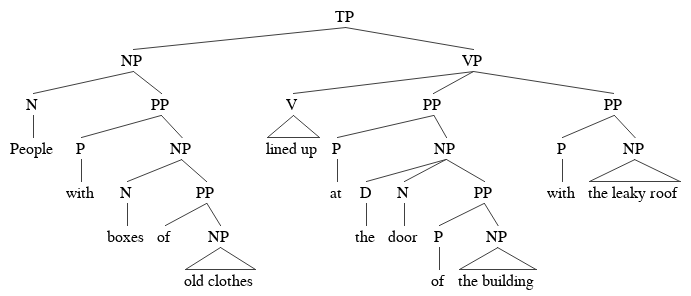[TP [NP [N People] [PP [P with] [NP [N boxes] [PP of [NP old clothes]]] ]][VP [V lined up] [PP [P at] [NP [D the] [N door] [PP [P of] [NP the building]] ]] [PP [P with] [NP the leaky roof]]]]

building with the leaky roof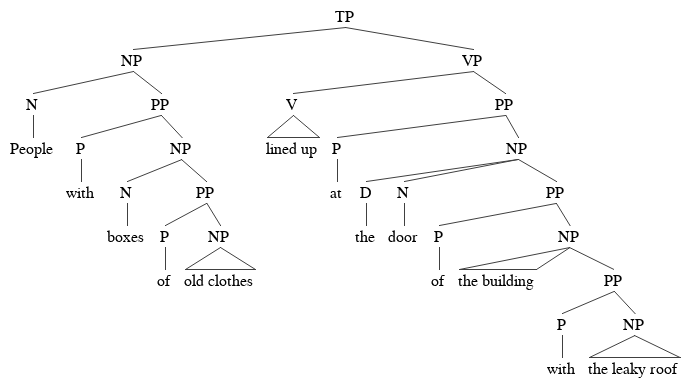[TP [NP [N People] [PP [P with] [NP [N boxes] [PP [P of] [NP old clothes]]] ]][VP [V lined up] [PP [P at] [NP the door]] [PP [P of] [NP the building [PP [P with] [NP the leaky roof]]]]]]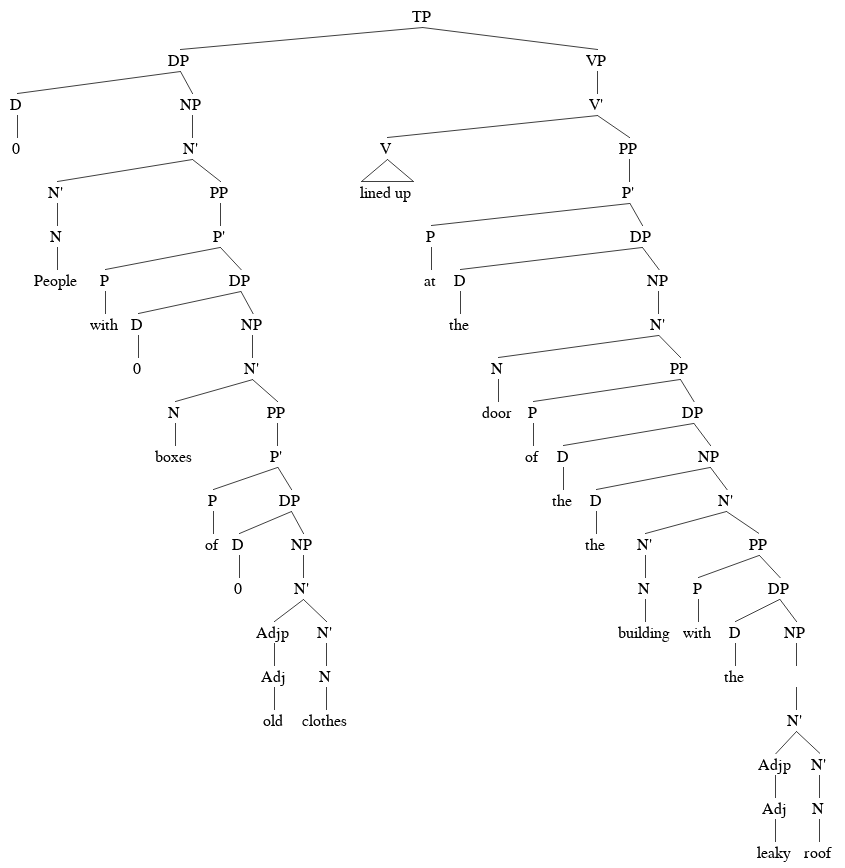[TP [NP [N People] [PP [P with] [NP [N boxes] [PP [P of] [NP old clothes]]] ]][VP [V lined up] [PP [P at] [NP the door]] [PP [P of] [NP the building [PP [P with] [NP the leaky roof]]]]]]

Final touches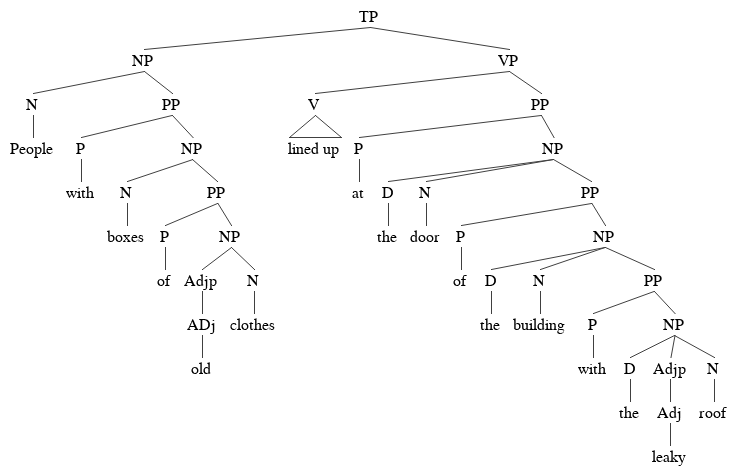[TP [NP [N People] [PP [P with] [NP [N boxes] [PP [P of] [NP [Adjp [ADj old ]] [N clothes]]]] ]][VP [V lined up] [PP [P at] [NP [D the] [N door] [PP [P of] [NP [D the] [N building] [PP [P with] [NP [D the] [Adjp [Adj leaky]] [N roof]]]]]]]]]

## Chapter 6

Chapter 6 tree for previous sentence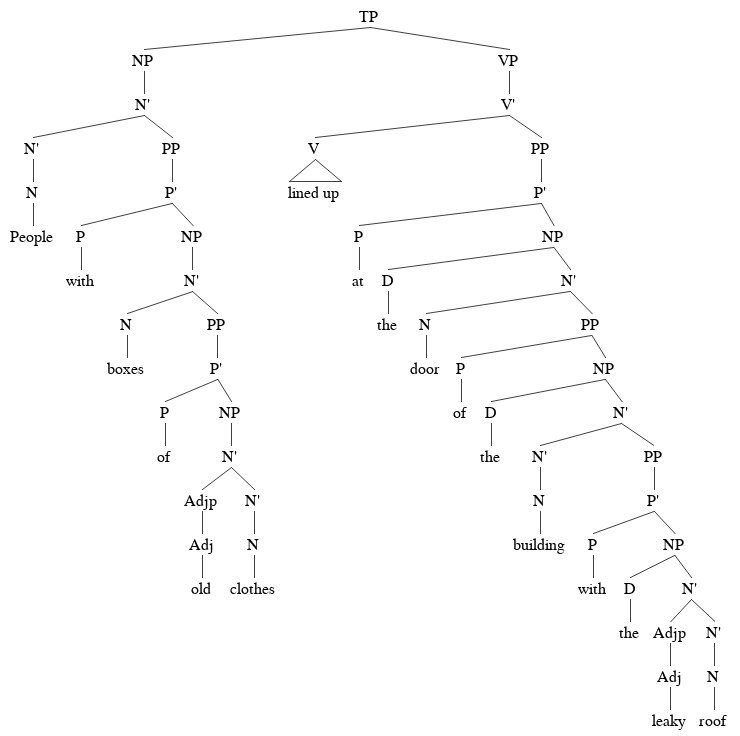[TP [NP [N' [N' [N People]] [PP [P' [P with] [NP [N' [N boxes] [PP [P' [P of] [NP [N' [Adjp [Adj old ]] [N' [N clothes]]]]] ]]]]] ]] [VP [V' [V lined up] [PP [P' [P at] [NP [D the] [N' [N door] [PP [P of] [NP [D the] [N' [N' [N building]] [PP [P' [P with] [NP [D the] [N' [Adjp [Adj leaky]] [N' [N roof]]]]]]]]]]]]]]]]

## Chapter 7

Continuing with the same sentence, we will turn it into a Ch. 7 tree. Let's add DPs first.

Step One: Add a DP nodes above every NP.Step Two: Add a determiner head to every DP as follows. If the NP didn't have a determiner add an empty determiner head. If the NP had a determiner, move the determiner out of the NP.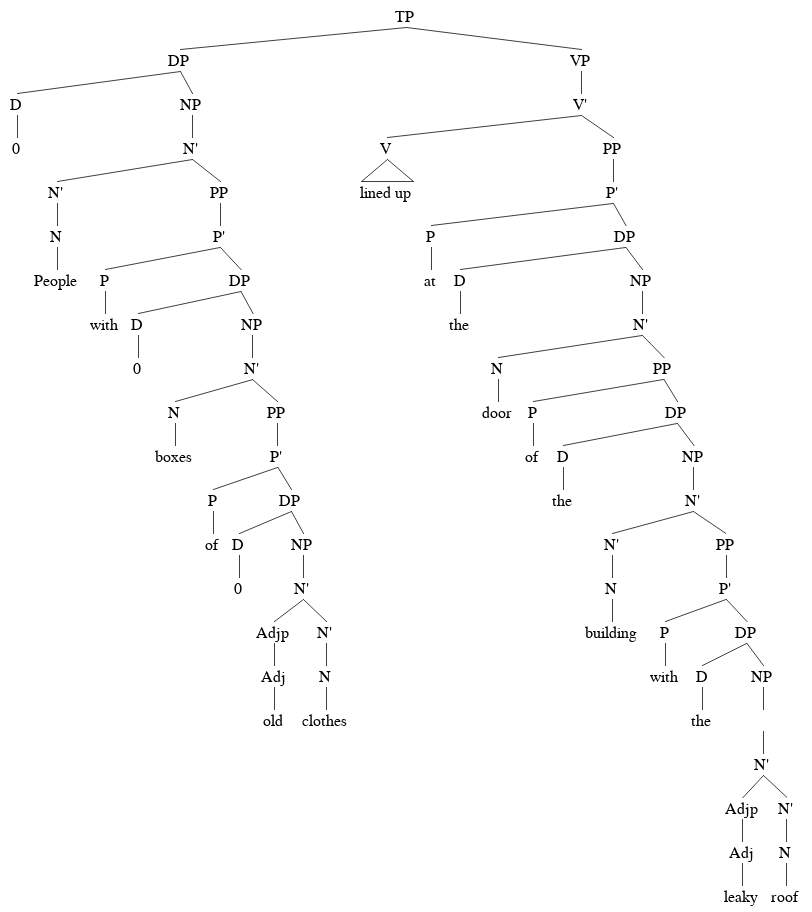[TP [DP [D 0] [NP [N' [N' [N People]] [PP [P' [P with] [DP [D 0] [NP [N' [N boxes] [PP [P' [P of] [DP [D 0] [NP [N' [Adjp [Adj old ]] [N' [N clothes]]]]] ]]]]] ]]]]] [VP [V' [V lined up] [PP [P' [P at] [DP [D the] [NP [N' [N door] [PP [P of] [DP [D the] [NP [N' [N' [N building]] [PP [P' [P with] [DP [D the] [NP [ [N' [Adjp [Adj leaky]] [N' [N roof]]]]]]]]]]]]]]]]]]]]
Step Three: Add intermediate level categories (D') to complete DPs.[TP [DP [D' [D 0][NP [N' [N' [N People]] [PP [P' [P with] [DP [D' [D 0] [NP [N' [N boxes] [PP [P' [P of] [DP [D' [D 0] [NP [N' [Adjp [Adj old ]] [N' [N clothes]]]]] ]]]]] ]]]]]]]] [VP [V' [V lined up] [PP [P' [P at] [DP [D' [D the] [NP [N' [N door] [PP [P of] [DP [D' [D the] [NP [N' [N' [N building]] [PP[P' [P with] [DP [D' [D the] [NP [ [N' [Adjp [Adj leaky]] [N' [N roof]]]]]]]]]]]]]]]]]]]]]]]

Add T', C', empty T, empty C, where necessary. The tree below is a fully correct Chapter 7 tree.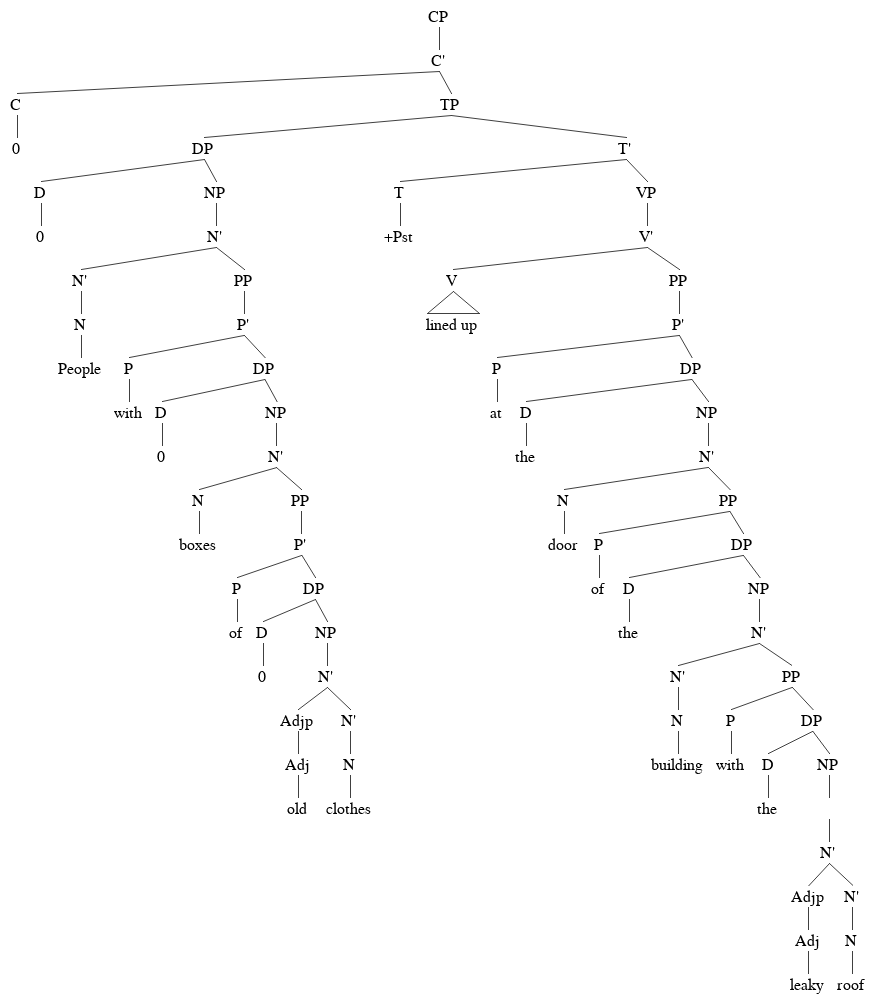[CP [C' [C 0] [TP [DP [D 0] [NP [N' [N' [N People]] [PP [P' [P with] [DP [D 0] [NP [N' [N boxes] [PP [P' [P of] [DP [D 0] [NP [N' [Adjp [Adj old ]] [N' [N clothes]]]]] ]]]]] ]]]]] [T' [T +Pst] [VP [V' [V lined up] [PP [P' [P at] [DP [D the] [NP [N' [N door] [PP [P of] [DP [D the] [NP [N' [N' [N building]] [PP [P with] [DP [D the] [NP [ [N' [Adjp [Adj leaky]] [N' [N roof]]]]]]]]]]]]]]]]]]]]]]

## Binding Theory Question

When we did the binding theory in Ch. 5, we had Chap 3 style trees. Suppose we were going to do the binding theory with Chapter 7 style trees. What nodes should we index? NP or DP? Assume the binding principles are the same as before. So for Prin A, an anaphor must be bound.

Consider the following example which should turn out to be grammatical. That is, the anaphor himself should turn out to be bound, as required by Prin A.

Which tree gets the right result?

Tree A: We index NPs.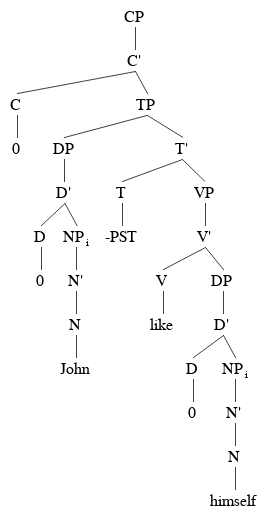Tree B: We index DPs.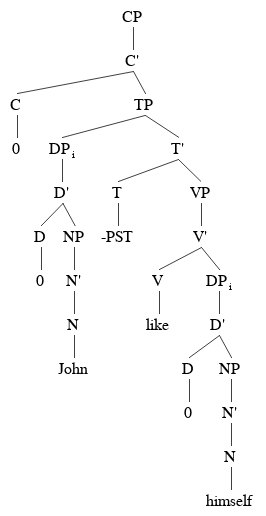## Embedded clauses in Chapter 7

A new example[CP[C' [C 0] [TP [DP [D'[D 0] [NP [N' [N I]]]] ][T' [T must] [VP [V' [V ask] [CP [C' [C 0] [TP [DP [D' [D 0] [NP [N' [N you]]]]] [T' [T to] [VP [V' [V leave]]]]]]]]]]]]]